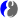# DMelt:Programming/3 Jython

Limitted access. First login to DataMelt if you are a full DataMelt member. Then login to HandWiki as a user.

# Using Jython/PythonJython is is an implementation of the Python programming language designed to run on the Java platform. See more resources here:

# Benchmarking Jython

Here you can find some benchmark results for Jython and BeanShell scripting. As explained in Section Running DMelt, one can execute macros/source files using the "run" button: DataMelt recognizes file formats on the fly.

Here is an example which benchmarks several arrays implemented in the native Java (ArrayList), Python (list) and DataMeltjhplot.P0D array. The script below checks the CPU time needed to fill these arrays with Gaussian-distributed random numbers:

from java.util import Random
from jhplot import *
import time

a=P0D("high-performance")
b=ArrayList()
c=[]
r=Random()

Ntot=3000000 # number of events for testing

start = time.clock()
for i in range(Ntot):
x=r.nextGaussian()
print ' CPU time for P0D (s) in Jython Loop=',time.clock()-start

start = time.clock()
a.randomNormal(Ntot, 0, 1)
print ' CPU time for P0D (s) native method =',time.clock()-start

start = time.clock()
for i in range(Ntot):
x=r.nextGaussian()
c.append(x)
print ' CPU time for Jython list (s)=',time.clock()-start

start = time.clock()
for i in range(Ntot):
x=r.nextGaussian()
print ' CPU time for Java ArrayList (s)=',time.clock()-start

A typical result (using G2030, Dell Insperon 660s) of this benchmark is shown below:

CPU time for P0D (s) in Jython Loop= 2.792909833
CPU time for P0D (s) native method = 0.390237158
CPU time for Jython list (s)= 2.443057156
CPU time for Java ArrayList (s)= 3.608499232

As you can see, the performance of P0D when using its native method is a factor 10 better.

# Benchmarking BeanShell

You can also use the BeanShell as an alternative scripting language. Generally, BeanShell is slower the Jython in loops, but if you call the native methods of high-performance classes (as for P0D in the example above), then the execution speed will be very similar:

import java.util.*;
import jhplot.*;
import time.*;

a=new P0D("high-performance");
b=new ArrayList();
r=new Random();

Ntot=3000000; ''  number of events for testing

start= System.currentTimeMillis();
for (i=0; i<Ntot; i++){
x=r.nextGaussian();
};

print("CPU time for P0D (s) in Jython Loop=");
print(0.001*(System.currentTimeMillis()-start));

start= System.currentTimeMillis();
a.randomNormal(Ntot, 0, 1);
print("CPU time for P0D (s) native  Loop=");
print(0.001*(System.currentTimeMillis()-start));

start= System.currentTimeMillis();
for (i=0; i<Ntot; i++){
x=r.nextGaussian();
};

print("CPU time for Java ArrayList Loop=");
print(0.001*(System.currentTimeMillis()-start));

Running the above code in the DataMelt gives:

bsh %
CPU time for P0D (s) in Jython Loop= 7.118
CPU time for P0D (s) native  Loop=0.377
CPU time for Java ArrayList Loop=7.131

# Benchmarking Groovy

You can also use Groovy as an alternative scripting language.

import jhplot.P0D

a=new P0D("high-performance")
r=new java.util.Random()

Ntot=3000000
start=System.currentTimeMillis();
for (i=0; i<Ntot; i++){
x=r.nextGaussian()
}
end_time=System.currentTimeMillis();
println  (end_time-start)/1000

The output printed in JRubyShell is

0.8

(0.8 seconds with a spread of 0.1)

# Benchmarking JRuby

You can also use JRuby as an alternative scripting language.

include_class Java::jhplot.P0D

a=P0D.new("high-performance")
r=java.util.Random.new()

Ntot=3000000 # number of events for testing
start=Time.now
for i in 1..Ntot
x=r.nextGaussian()
puts "Time elapsed #{(end_time - start)} seconds"
Time elapsed 0.8 seconds